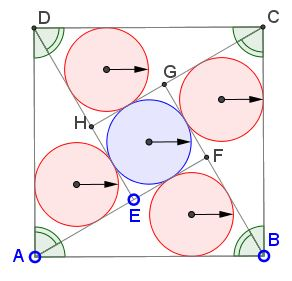## Five Incircles in a Square: What Is This About? A Mathematical Droodle

Created with GeoGebra

Explanation### Five Incircles in a Square

Here we have a very characteristic sangaku - a Temple geometry - problem. Most of geometric sangaku dealt with several circles inscribed in other circles or other shapes. In addition, most often a sangaku was a computational problem as opposed to problems that require a proof. The problem below is a little of both: a proof of the result is supported by a chain of calculations.

In the manner of proof #3 of the Pythagorean theorem, four equal right triangles and a small square are combined into a larger square. Circles are inscribed into the four triangles and the inner square. A question: What can be said of the configuration where all five circles have equal radii?So assume the triangles have legs a and b (a > b) and hypotenuse c. Then, as we know, the inradius for the triangle is given by

r = (a + b - c)/2.

On the other hand, the radius of the circle inscribed into the inner square is obviously

r = (a - b)/2.

Equating the two we get

c = 2b,

which means that the angle opposite b is 30° and the other one is 60°. From here we easily find the common inradius: r = c·(3 - 1)/4.

Most of the sangaku problems are much more difficult.

### References

1. H. Fukagawa, D. Pedoe, Japanese Temple Geometry Problems, The Charles Babbage Research Center, Winnipeg, 1989

Write to:

Charles Babbage Research Center
P.O. Box 272, St. Norbert Postal Station
Winnipeg, MB